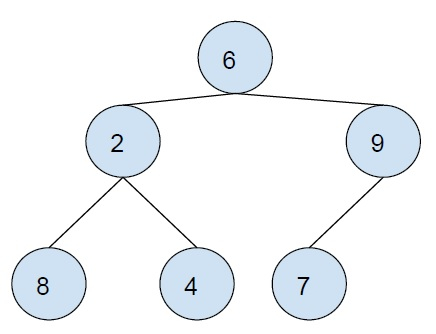# Postorder successor of a Node in Binary Tree in C++

In this problem, we are given a binary tree and node. Our task is to print the postorder successor of the node in Binary tree.

Binary tree is a special type of tree in which each node can have at max 2 child nodes.Postorder Traversal is a tree traversal technique, in which the first left subtree is traversed than the right subtree and the root is traversed at the end.

postorder traversal of the above tree: 8 4 2 7 9 6

Let’s take an example to understand the problem.

Input − binary tree in above example, node= 7

Output − 9

Explanation − we can see it in the post-order traversal of the binary tree.

To solve this problem, a simple, easy and noob’s solution will be simply finding the postorder traversal and then print the number next to it in the traversal.

But we need to learn a more effective solution,

An effective solution will involve using some general observation on the postorder traversal.

• Since the root is the last node in postorder traversal, its successor is NULL.

• In the case of the current node being the right child, the parent node is a successor.

• In case of current node being left child, then

• If the right sibling is absent, the successor is a parent node

• If the right sibling exist then either the node or its leftmost child is successor.

This method is effective, the time complexity is O(h), his height of tree.

## Example

Program to show the implementation of our solution,

Live Demo

#include <iostream>
using namespace std;
struct Node {
struct Node *left, *right, *parent;
int value;
};
struct Node* insertNode(int value) {
Node* temp = new Node;
temp->left = temp->right = temp->parent = NULL;
temp->value = value;
return temp;
}
Node* findPostorderSuccessor(Node* root, Node* n) {
if (n == root)
return NULL;
Node* parent = n->parent;
if (parent->right == NULL || parent->right == n)
return parent;
Node* curr = parent->right;
while (curr->left != NULL)
curr = curr->left;
return curr;
}
int main(){
struct Node* root = insertNode(6);
root->parent = NULL;
root->left = insertNode(2);
root->left->parent = root;
root->left->left = insertNode(8);
root->left->left->parent = root->left;
root->left->right = insertNode(4);
root->left->right->parent = root->left;
root->right = insertNode(9);
root->right->parent = root;
root->right->left = insertNode(7);
root->right->left->parent = root->right;
root->left->right->left = insertNode(14);
struct Node* successorNode = findPostorderSuccessor(root, root->left->right);
if (successorNode)
cout<<"Postorder successor of "<<root->left->right->value<<" is "<<successorNode->value;
else
cout<<"Postorder successor of "<<root->left->right->value<<" is NULL";
return 0;
}

## Output

Postorder successor of 4 is 2